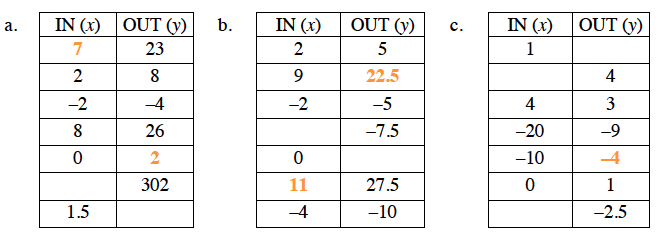### Home > CC3MN > Chapter 3 Unit 3 > Lesson CC3: 3.1.1 > Problem3-4

3-4.

For each $x$$y$ table given, copy the table, find the pattern and fill in the missing entries. Then write the rule for the pattern in words. Homework Help ✎

1.  IN ($x$) OUT ($y$) 23 2 8 –2 –4 8 26 0 302 1.5 Rule:
1.  IN ($x$) OUT ($y$) 2 5 9 –2 –5 –7.5 0 27.5 –4 –10 Rule:
1.  IN ($x$) OUT ($y$) 1 4 4 3 –20 –9 –10 0 1 –2.5 Rule:

Refer to problem 3-3 that you completed in class.

Here are some answers to get you started.Rule: Triple $x$ and add $2$.